# Band of semi-groups

of a given familyA semi-groupthat has a partition into sub-semi-groups whose (isomorphism) classes are just the semi-groups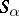, and such that for any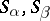there is an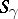such that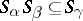.is also said to be decomposable into the band of semi-groups. In other words,has a partition into a band of semi-groupsif all theare sub-semi-groups ofand if there is a congruenceonsuch that the-classes are just the. The semi-groupsare called the components of the given band. The term "band of semi-groups" is consistent with the frequent use of the word "band20M14band" as a synonym of "semi-group all elements of which are idempotents" , since a congruenceon a semi-groupdetermines a partition ofinto a band if and only if the quotient semi-group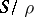is a semi-group of idempotents.

Many semi-groups are decomposable into a band of semi-groups with one or other "better" property; thus, the study of their structure is reduced in some measure to a consideration of the types to which the components of a band belong, and of semi-groups of idempotents (see, e.g. Archimedean semi-group; Completely-simple semi-group; Clifford semi-group; Periodic semi-group; Separable semi-group).

A band of semi-groupsis said to be commutative if for the corresponding congruencethe quotient semi-groupis commutative; thenis a semi-lattice (in this case,is frequently called a semi-lattice of semi-groups; in particular, ifis a chain, thenis called a chain of semi-groups). A band of semi-groups is called rectangular (sometimes matrix) ifis a rectangular semi-group (see Idempotents, semi-group of). Equivalently, if the components of the band can be indexed by pairs of indices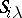, whereandrun over certain setsand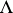, respectively, such that for any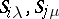one has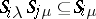. Any band of semi-groups is a semi-lattice of rectangular bands, that is, its components can be arranged into subfamilies so that the union of the components of each subfamily is a rectangular band of components, and the original semi-group is decomposable into a semi-lattice of these unions (Clifford's theorem ). Since the properties of being a semi-group of idempotents, a semi-lattice or a rectangular semi-group are characterized by identities, for each of the listed propertiesthere is a finest congruence on any semi-groupfor which the corresponding quotient semi-group has the property, that is, there exist greatest (or biggest quotient) partitions ofinto a band of semi-groups, into a commutative band of semi-groups and into a rectangular band of semi-groups.

The term strong band concerns special types of bands of semi-groups : For any elements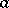and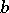from different components, the product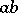is a power of one of these elements. An important special case of a strong band, and also a special case of a chain of semi-groups, is the ordinal sum (or sequentially-annihilating band): The set of its componentsis totally ordered, and for anysuch that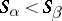, and for any,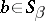one has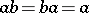; the ordinal sum is defined uniquely up to an isomorphism, by specifying the components and their ordering.

How to Cite This Entry:
Band of semi-groups. L.N. Shevrin (originator), Encyclopedia of Mathematics. URL: http://encyclopediaofmath.org/index.php?title=Band_of_semi-groups&oldid=18665
This text originally appeared in Encyclopedia of Mathematics - ISBN 1402006098